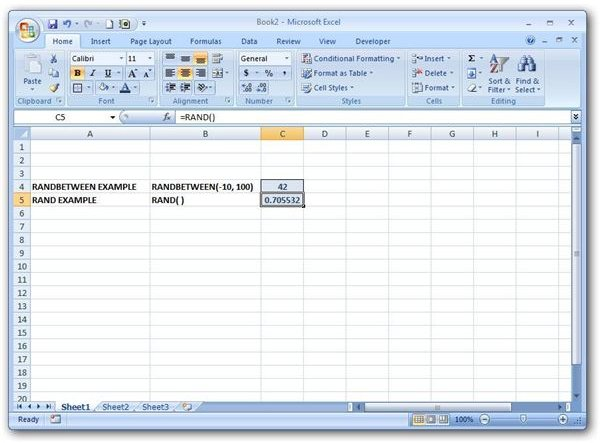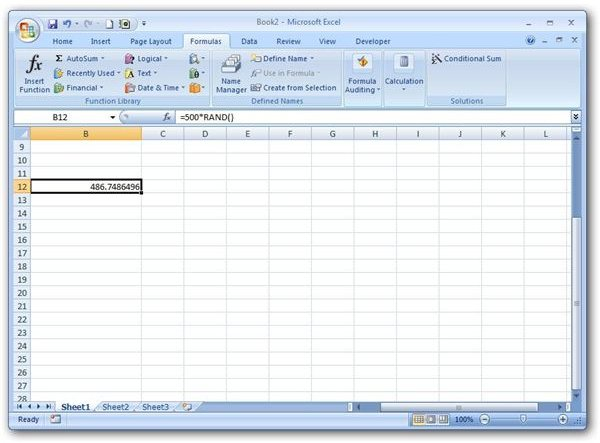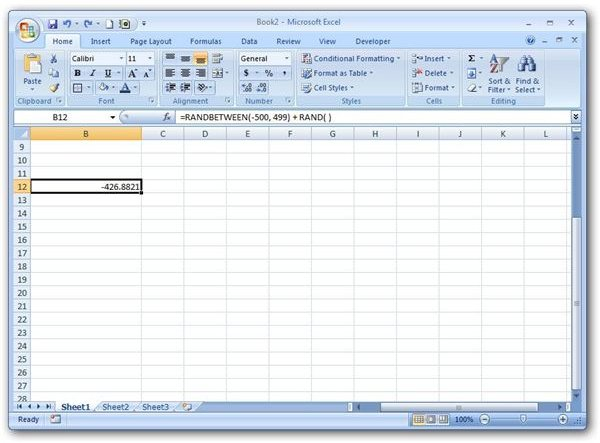# Random Number Generators in Excel: RAND and RANDBETWEEN Functions

Page content

## Excel Random Number Generators

There are two basic functions that can be used to generate a random number in Microsoft Excel: RAND and RANDBETWEEN. The RANDBETWEEN function is more customizable, in a sense, because you can choose a lower and an upper limit for the random numbers that are generated. However, all values produced by this function are of integer form. If you want to be able to generate any real number, it’s best to use a formula containing the RAND function.

## Function Syntax and Use

The basic syntax for the RANDBETWEEN function is

RANDBETWEEN(lower, upper)

where lower is the smallest integer that will be returned by the function and upper is the largest number. For example, the function

RANDBETWEEN(-10, 100)

will return an integer value that is greater than or equal to -10 and less than or equal to 100. (Click any image for a larger view.)

The syntax for the RAND function is much simpler as it contains no arguments:

RAND( )

This function will return a real number that is greater than or equal to 0 but less than 1.Note that each time the spreadsheet is recalculated, the values of these functions will change. So, if you want the evaluation to be fixed, it is best to set Excel’s Automatic Calculation feature to Manual. For more information on how to do this in Excel 2007, see the article Automatic Calculation Options in Excel 2007.

## Function Examples

Example 1: What if you want to generate a real number, but you want to include possibilities greater than 1? That is, let’s suppose we want to produce a random real number between 0 and 500, including 0 but not 500. We can still use the RAND function, but our formula will also have to include an additional multiplier. Since RAND will generate a number between 0 and 1, multiplying the result by 500 will give us a random number between 0 and 500. The resulting function would be the following.

=500*RAND( )Example 2: Now, suppose that we want to produce a random real number between -500 and 500, including -500 but not 500. We can’t use the same method as we did in Example 1, since simply multiplying by a constant number would give us a value between 0 and that constant. Instead, we can create a formula that combines the RAND and RANDBETWEEN functions.

We know that the function RANDBETWEEN(-500, 499) will produce a random integer in our chosen range, but we want to be able to produce any real number, not just integers. We can accomplish this by adding RAND() to the prior function to obtain the following formula.

=RANDBETWEEN(-500, 499) + RAND( )Additional Resources: For more tips and tricks, take a look at the ever-growing collection of Microsoft Excel user guides and tutorials available on Bright Hub’s Windows Channel. Learn design tips for various charts and graphs, how to use the LOOKUP function to query a table, how to create a dropdown list, and more. More resources are being added all the time so be sure to keep checking back.